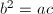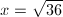# Algebra: What is mean Proportion and How to solve it?

A proportion such as 1:2 =2:4 or a:b = b:c  is called a mean proportion because the second and the third terms are equal. In the latter b is the mean proportional between the first and the last terms a and c, respectively and c is the third proportional to a and b.

Solving for the mean proportionalGivenin proportion, the product of the extremes is equal to the product of the means.extracting the square roots of booth members of the equation

Note; like roots and like powers of equal numbers are equal

It follows that:
The mean proportional between two quantities is equal to the square root of their product.

example 1 :
Solve for x inexample 2 :
Solve for x in

# Algebra: How to solve the Equations containing decimals

Equations containing decimals are equations with fractions. hence the solution of decimal equations is similar to that of fractional equations with this difference-in decimal equation the multiplier of both members is that power
of ten that will clear the equations of its decimal.

Example1.
Solve for x:                                 .3x + 5 = .2x
Multiplying both members
of the equation by 10,                 3x + 50 = 2x
x = -50

Check                                     .3x(-50) + 5 = .2(-50)
-15+5  = -10
-10 = -10

Example no 2.                                      Solve for y in the equation
.5y/3 - .18/4 = .1y/6
Clear the equation of decimals by multiplying both members by 100
50y/3 - 18/4 = 10y/6
Clear the equation of fractions by multiplying both members by 12, the LCD
200y - 54 = 20y
180y = 54
y = 54/180 = .3

check:
.5y/3 - .18/4 = .1y/6
(.5(.3))/3 -.18/4  = (.1(.3))/6
.05-.045 = 0.005
.005 = .005